WEBINAR
Numerical Analysis of Magnetic Pulse Welding Process
Thursday, October 5, 2023
Time
SESSION 1
SESSION 2
CEST (GMT +2)
03:00 PM
08:00 PM
EDT (GMT -4)
09:00 AM
02:00 PM
HOME / Applications / Force in a magnetic circuit

# Force in a magnetic circuit

Used Tools:## Physics

A magnetic circuit is a physical system whose components produce and contain magnetic flux. Flux is generally produced by permanent magnets or coils, while the flux paths consist of some ferromagnetic material of high permeability, such as iron. The example of magnetic circuit  in Figure 1 contains two iron parts of cross-section area  . One part is stationary and the other is moving; they are separated by two air gaps of length $\mathrm{L. N}$-turn coil wrapped around the stationary part, carries the current I ( ) and is responsible for the flux in the circuit.

Force on the moving part can be determined by calculating the change in the magnetic energythat would be produced by moving the part over a small distance. Force magnitude is obtained as:(eq.1)

This simple method is based upon the virtual displacement principle and it is often used to calculate forces in magnetic devices.
Since the permeability of iron is much greater than the permeability of air (), magnetic field in the iron (), as well as leakage flux, are neglected. Ampere’s law for the contour in Figure 1. can be written as:(eq.2)

where ${\mathrm{Ha}}_{}$  represents magnetic field in the air gap.

With no leakage and no filed in the iron (), the entire magnetic energy of the system is stored in the volume  of the air gap and can be computed as:(eq. 3)

Assuming uniform field distribution in the air gap allows to calculate $W$ by simply multiplying energy densityand volume $V$. The circuit contains 2 air gaps, therefore, after doubling the energy in eq.3 and substituting eq.2 in eq.3:(eq. 4)

For two different air gap lengths  ${\mathrm{L1}}_{}=1.5mm$  and ${\mathrm{L2}}_{}=2.5mm$ , change in magnetic energy can be computed as:(eq. 5)

Equation 5, combined with eq. 1, yields the following expression for force magnitude:(eq. 6)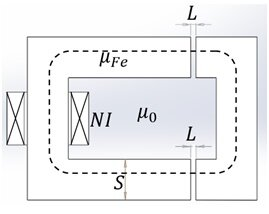Figure 1 - Magnetic circuit example

## MODEL

To accurately represent situation in the analytical example, where force has been computed using energies for  $1.5mm$  and $2.5mm$ air gaps, Solidworks model of the circuit has been created with two air gaps of different lengths: and ${\mathrm{L2}}_{}=2.5mm$ (Figure 2.).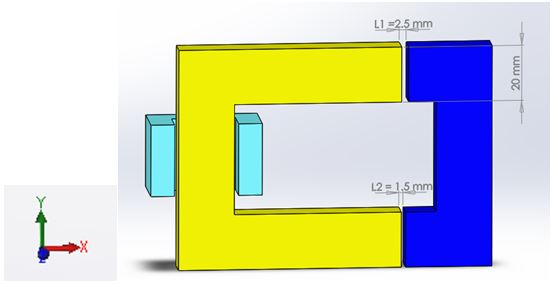Figure 2 - Model of one half of the magnetic circuit is created in SOLIDWORKS

Due to the symmetry of the problem, it is enough to simulate only one half of the space (half of the magnetic circuit). The simulation uses EMS Magnetostatic study, with symmetry factor of  0.5 .
Current driven Wound Coil with $300$ turns and $1A/turn$ is added to the coil region. Its Entry and Exit Ports are faces in the plane of symmetry.

## Material

The following instructions show you how to create new material library, define custom material and add material to an element of your model:

1. In the EMS Manager tree, right-click the Stationary element icon in the Materials folder and select Apply Material to All Bodies. The Material Task Pane opens.
2. Select “New Library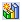from the “Material Task Pane”.
3. Browse to the location where to save the new library file (the file extension will be .emsmtr).
4. Type MyLib for the name. An empty material library with the MyLib is added to the Material Task Panel.
5. Click on Add Materiel button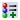.
6. Type Mur1200 for the material's name.
7. Click OK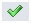.
8. Type 1200 for the Relative Permeability and take the default for the rest of the fields.
9. Click OK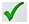.

Copper is assigned to the coil region, and air to the surrounding volume.

## Boundary Conditions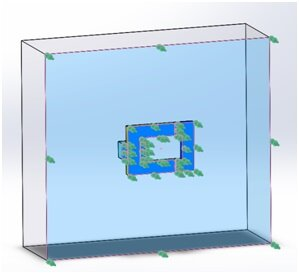Figure 3 -
Tangential Flux boundary condition

Normal component of the flux density at the plane of symmetry ( $xy$ plane in Figure 2.) is zero (all flux lines are parallel to this plane). Therefore, Tangential Flux boundary condition should be applied on all surfaces that belongs to the plane, including air and coil cross-sections. To do so:

1. In the study, right-click the Load/Restraint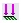folder and select Tangential Flux. The Tangential Flux Property page appears.
2. Click inside the Faces for Tangential Flux box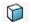then select all the symmetry faces.
3. Click OK.

## Coils

To add a coil to a Magnetostatic study:

1. In the EMS manger tree right-click on the Coils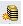icon and select Wound Coil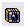.
2. Keep default Coil Type as a Current driven coil.
3. Click inside the Components or Bodies for Coils box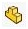.
4. Click on the (+) sign in the upper left corner of the graphics area to open the components tree.
5. Click on the Coil-1 icon.  It will appear in the Components and Solid Bodies list.
6. Click inside the Faces for Entry Port box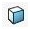then select the entry port face.
7. Click inside the Faces for Exit Port boxthen select the exit port face.
General Properties:
1. Click on General properties tab.
2. Type 300 in the Turns box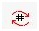.
3. Keep the default value of 1 the Current per Turn  field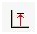. The units in Amp-Turns.
4. Click OK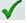.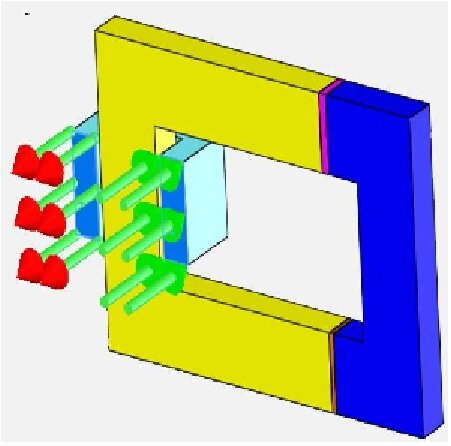Figure 4 - Entry and exit ports of the coil

## Force

EMS automatically computes the nodal force distribution without any user input.  However, for a rigid body force calculation, part on which the force or torque shall be calculated needs to be defined before running the simulation.

1. In the EMS manager tree, right-click the Force/Torques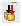folder and select Virtual Work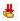.
2. The Forces/Torques Property Manager appears.
3. Click inside the Components and Bodies for Forces/Torques box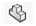.
4. Click on the (+) sign in the upper left corner of the graphics area to open the components tree.
5. Click on Moveable part icon.  It will appear in the Components and Solid Bodies list.
6. Click OK.

## Mesh

Quality of the mesh in the air gap region is of critical importance for accurate force calculation. EMS allows user to take full control over the mesh resolution.

1. In the EMS manager tree, right-click the Mesh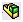icon and select Apply Control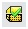. The Mesh Control Property Manager Page appears.
2. Click inside the Components and Solid Bodies box.
3. Click on the (+) sign in the upper left corner of the graphical area to open the components tree.
4. Click on the Air Gap 1, and Air Gap 2 icons.  They will appear in the Components and Solid Bodies list.
5. Under Control Parameters click inside the Element Size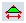box and type 2.5 mm.
6. Click OK.

To mesh the model:

1. In the EMS manger tree, right-click on the Mesh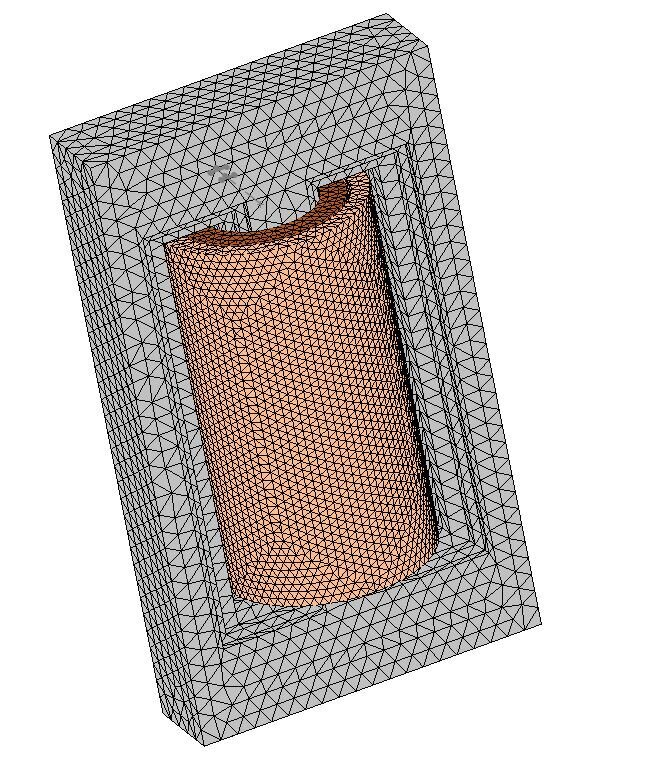icon and select Create Mesh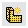.
2. Type 35 in the box for average number of mesh elements per diagonal for each solid body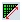.
3. Click OK.

Right-click Study icon and selected Run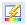, to execute simulation. Once the computation is done, the program creates five folders in the EMS manager tree. These folders are: Report, Magnetic Flux density, Magnetic Field intensity, Current density, and Force Distribution.

## RESULTS

### Flux density

It is a good habit to first view the magnetic flux density in the model, including the outer air.  This action gives an indication whether the outer air boundary is far enough.

1. In the EMS Manager tree, right-click on the Magnetic Flux Density folder and select 3D Fringe Plot> No Clipping.The Magnetic Flux Density Property Manager Page appears.
2. In the Display box:
a. Select Br from the magnetic flux density component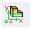.  Directions are based on the global coordinate system.
b. Set Units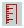to Tesla.
c. Select Continuous from Fringe Options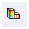.

3. Select OK.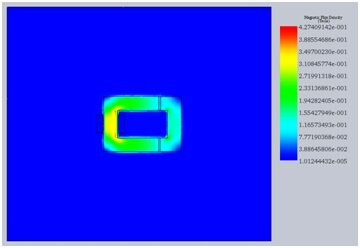Figure 5 - Flux density in the magnetic circuit

By examining Figure 5. it becomes clear that the magnetic flux density is very small on the outer air boundary.  Thus, the air box is large enough.  Had it been otherwise, air box surrounding the magnetic circuit would have to be larger.

## Force

In the EMS Manager tree, Double-click Result Table to display force result.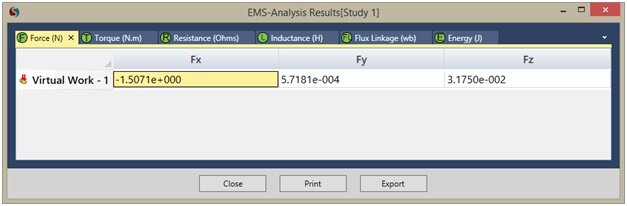Figure 6 - EMS Results Table

Remember that, because of the symmetry about xy plane, only half of the problem has been modeled. Thus, $\mathrm{Fx}$ and  components must be multiplied by a factor of 2, while the $\mathrm{Fz}$ component cancels out. Since $\mathrm{Fy}$ is very small compared to $\mathrm{Fx}$ , the resultant force is virtually in the X direction with a magnitude of  . Analytical solution compares very well with the EMS result.

 Analytical Virtual Work Solution EMS Result Force [N] 3.02 3.014

## References

 Electromagnetics and calculation of fields, by Nathan Ida and Joao P. A. Bastos, 2nd Edition, page 183-184.  Publisher: Springer-Verlag;